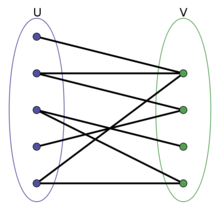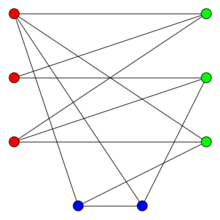### 输入输出样例

3 3
1 2
1 3
2 3


Impossible


3 2
1 2
2 3


1

### 说明/提示

【数据规模】

1<=N<=10000，1<=M<=100000，任意两点之间最多有一条道路。

### 题解1 #include <iostream>
2 #include <math.h>
3 #include <stdio.h>
4 #include <algorithm>
5 #include <string.h>
6
7 using namespace std;
8
9 const int MAXN = 100005;
10 int first[MAXN], n, m, en, color[MAXN], f, u, v; //f统计不同颜色节点数
11 int front, rear;
12 bool vis[MAXN];
13 int ans;
14
15 struct edge
16 {
17     int zhongdian, changdu;
18     int next;
19 };
20
21 edge ed[MAXN];
22
23 void add_edge(int s, int e, int d)
24 {
25     en++;
26     ed[en].next = first[s];
27     first[s] = en;
28     ed[en].zhongdian = e;
29     ed[en].changdu = d;
30 }
31
32 struct Node
33 {
34     int x, y;
35     int step;
36 };
37 Node q[MAXN];
38
39 int bfs(int a)
40 {
41     Node now, next;
42     now.x = a;
43     vis[a] = 1;
44     color[a] = 1;
45     f = 0;
46     f = 1;
47     front = rear = 0;
48     q[rear] = now;
49     rear++;
50     while(front < rear)
51     {
52         now = q[front++];
53         for(int i = first[now.x]; i; i = ed[i].next)
54         //first[now]：当前点的第一条边；ed[i].next：下一个访问的点
55         {
56             if(color[ed[i].zhongdian] != -1)
57             {
58                 if(color[ed[i].zhongdian] == color[now.x])
59                 {
60                     cout << "Impossible" << endl;
61                     return -1;
62                 }
63             }
64             else
65             {
66                 color[ed[i].zhongdian] = (color[now.x] + 1) % 2;
67                 q[rear].x = ed[i].zhongdian;
68                 rear++;
69                 f[color[ed[i].zhongdian]]++;
70             }
71         }
72     }
73     ans += min(f, f);
74 }
75
76 int main()
77 {
78     cin >> n >> m;
79     for(int i = 1; i <= n; i++)
80     {
81         color[i] = -1; //初始化
82     }
83     for(int i = 1; i <= m; i++)
84     {
85         cin >> u >> v;
88     }
89     for(int i = 1; i <= n; i++)
90     {
91         if(color[i] == -1)
92         {
93             if(bfs(i) < 0)
94             {
95                 return 0;
96             }
97         }
98     }
99     cout << ans << endl;
100
101     return 0;
102 }

posted on 2019-08-23 11:38  zealsoft  阅读(...)  评论(...编辑  收藏